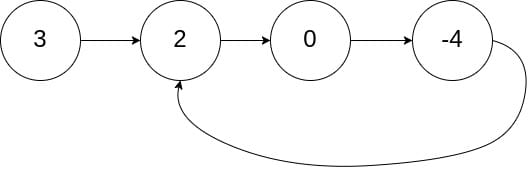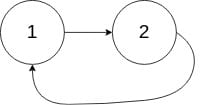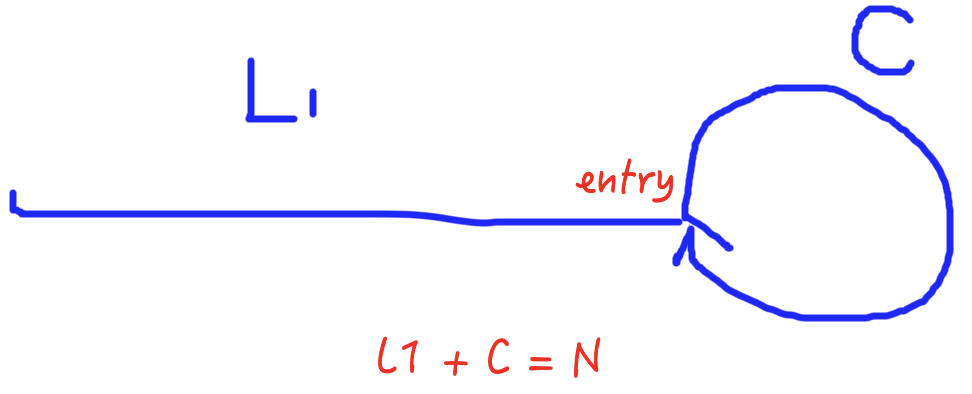Reference: LeetCode
Difficulty: EasyTwo Pointers

## Problem

Given a linked list, determine if it has a cycle in it.

Note:

• To represent a cycle in the given linked list, we use an integer pos which represents the position (0-indexed) in the linked list where tail connects to. If pos is -1, then there is no cycle in the linked list.
• There could be duplicates.

Example:Follow up: Can you solve it using $O(1)$ (i.e. constant) memory?

• Yes. Use two pointers or modify the next.

## Analysis

Note: In terms of duplicate nodes, they are different node objects. So it is okay to use hashCode() and ==.

Test Case:

### Hash Set

Use Set<ListNode> instead of Set<Integer>.

Time: $O(N)$.
Space: $O(N)$.

### Two Pointers

The space complexity can be reduced to $O(1)$ by considering two pointers at different speed. A slow pointer and a fast pointer. The slow pointer moves one step at a time while the fast pointer moves two steps at a time. If there is no cycle in the list, the fast pointer will eventually reach the end and we can return false in this case.• No cycle
The fast pointer reaches the end first and the run time depends on the list’s length, which is $O(N)$.
• Cycle exists
• Non-cyclic part:
• The slow pointer takes non-cyclic length steps to enter the cycle. The length is $L1$.
• Cyclic part:
• When two pointers enter into the cycle, it will take:
• (distance between two pointers (at most $C$) / difference of speed) moves for the fast pointer to catch up with the slow runner.

Therefore, the worst case runtime is $O(L1 + C)$, which is $O(N)$.

Why will the fast pointer eventually meet the slow pointer?

Consider the one-step case:

If the fast pointer is two steps behind the slower runner, this case will change into the one-step case above (each time the distance decreases by $1$):

Note: So many corner cases.

My originally bad code (using flag):

Improvement:

Time: $O(N)$
Space: $O(1)$

### Mark Node

By Argondey: I used a slightly faster destructive approach. I created a new node called “mark” and iterated through the list, setting the next value of each node to the mark node. When reaching the new node the first thing I did was check if the node is the mark node. If it ever is the mark node, we have looped. Brilliant!

Time: $O(N)$
Space: $O(1)$

CommentJunhao Wang
a software engineering cat